Associative property of multiplication

Basic multiplication is a pairwise operation. To multiply more than two numbers, you must combine them in pairs successively until all the numbers have been used. For example, to multiplyyou first pick two consecutive numbers, say 3 and 4, and multiply them: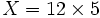. Now there are just two numbers remaining, which you can multiply to get the final answer: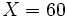.
The associative property of multiplication is the fact that the answer does not depend on how the pairings are done. For example, we could have started with, and then done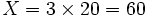. We use parentheses to indicate the order of multiplication used. For example, writingmeans that you first multiplythen multiply the result by 5. The associative property is expressed by the formula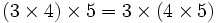.
With more than three numbers, there can be many ways to do the multiplication. For example, with 4 numbers one of the ways is.
The associative property of multiplication is different from the commutative property. For example, multiplication of matrices has the associative property but is not commutative. If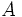,, and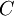are three matrices, then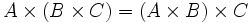but.